# Exponential and Logarithmic Functions

This post is part of a series.

Exponential functions have variables as exponents, e.g. $f(x)=2^x$.

Their end behavior consists of growing without bound to infinity in one direction, and decaying to a horizontal asymptote of $y=0$ in the other direction.

The size of the number that is exponentiated, called the base, governs which direction corresponds to which end behavior.

## Exponential Growth

If the magnitude of the base is bigger than $1$, then as $x$ increases, the function is repeatedly multiplied by a number bigger than $1$ and consequently grows without bound to infinity. For this reason, such functions are called exponential growth functions.

By the same token, as $x$ decreases, the function is repeatedly divided by a number bigger than $1$ and consequently decays to a horizontal asymptote of $y=0$.

For example, for the exponential growth function $f(x)=x^2$, each unit increase in $x$ causes the output to be doubled, and each unit decrease in $x$ causes the output to be halved.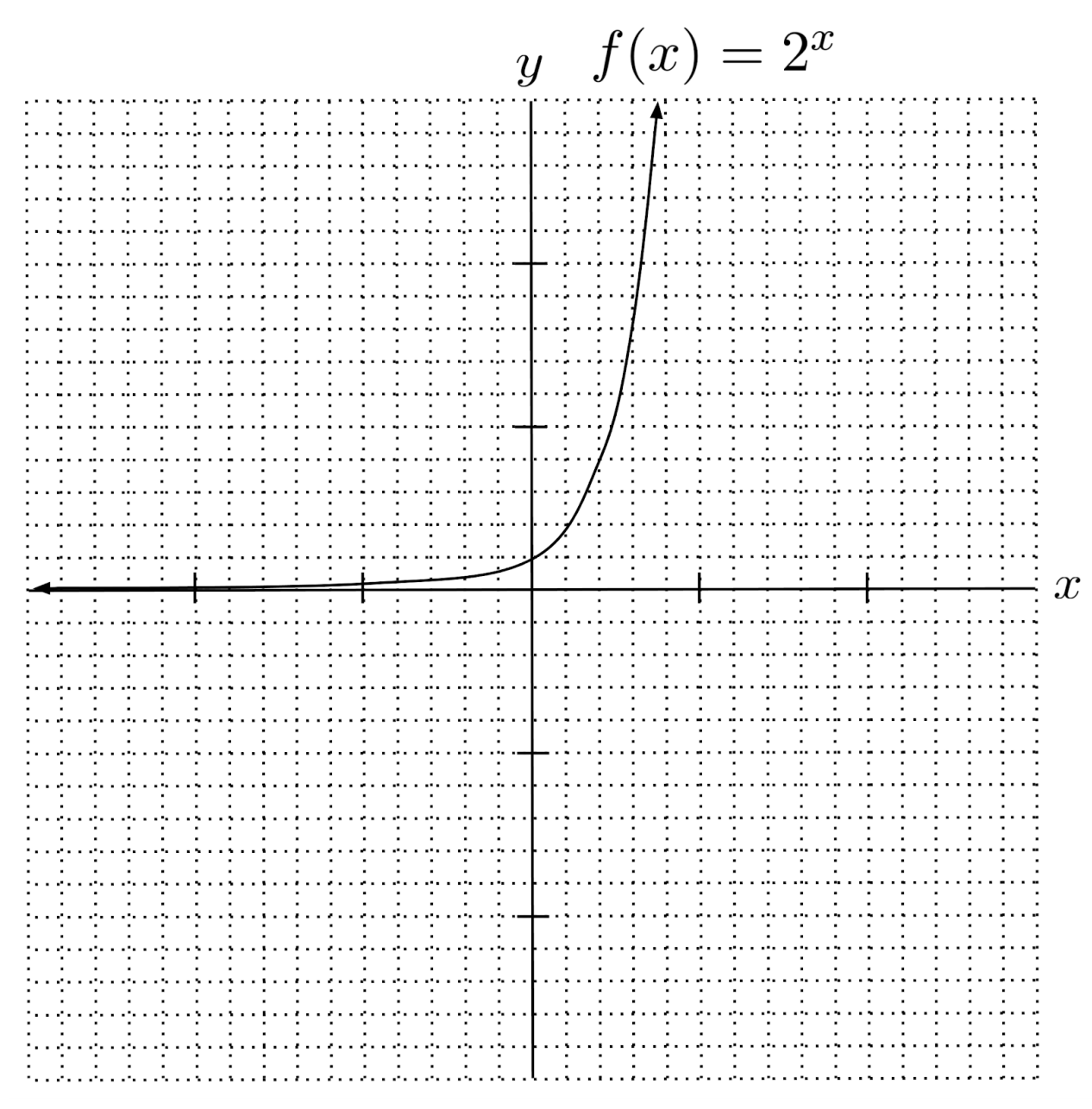## Exponential Decay

On the other hand, if the magnitude of the base is smaller than $1$, then as $x$ increases, the function is repeatedly multiplied by a number smaller than $1$ and consequently decays to a horizontal asymptote of $y=0$. For this reason, such functions are called exponential decay functions.

By the same token, as $x$ decreases, the function is repeatedly divided by a number smaller than $1$ and consequently grows without bound to infinity.

For example, for the exponential decay function $f(x) = \left( \frac{1}{2} \right)^x$, each unit increase in $x$ causes the output to be halved, and each unit decrease in $x$ causes the output to be doubled.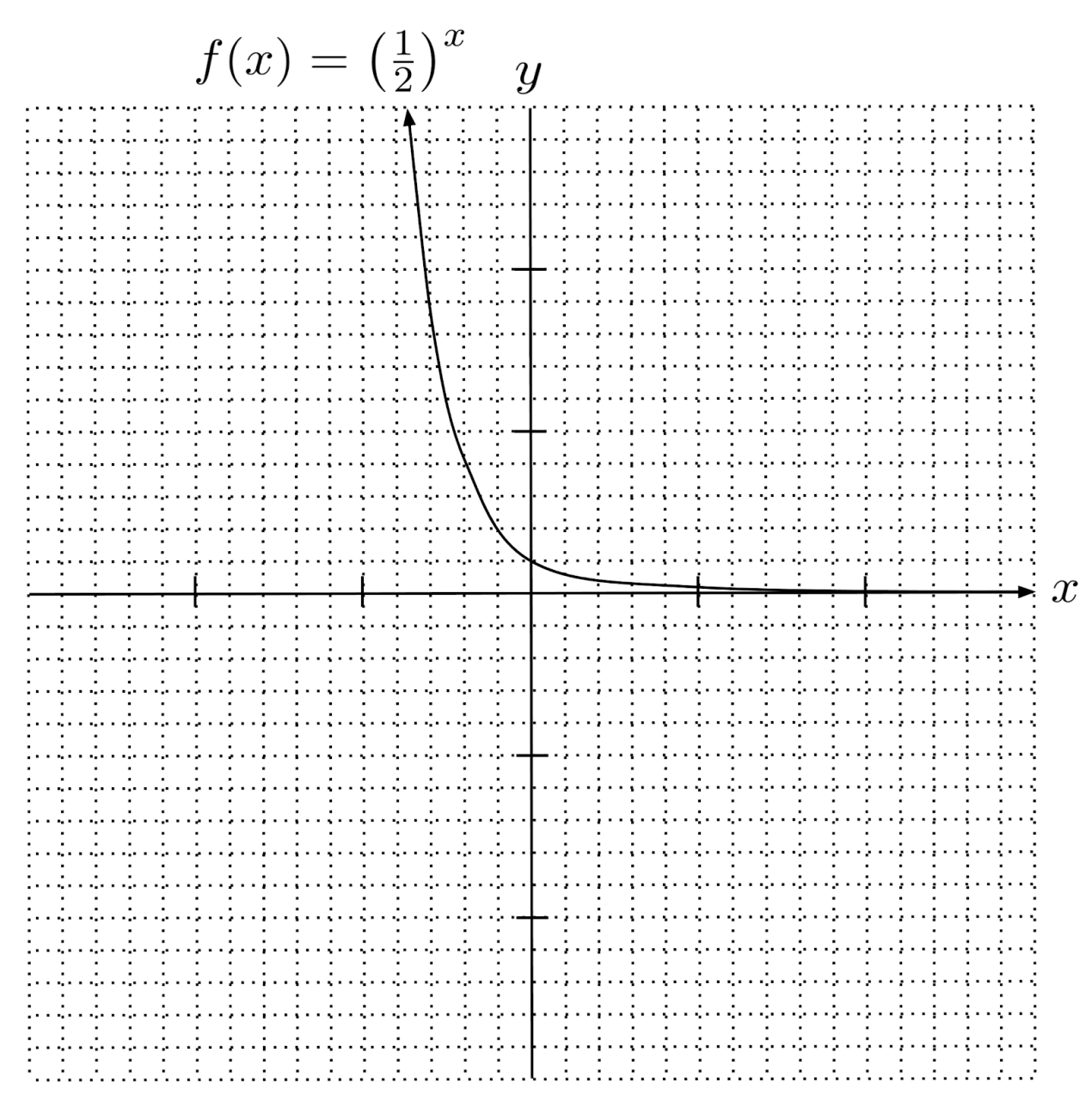## Logarithms

Equations involving exponential terms can be solved with the help of logarithmic functions, which cancel out exponentiation.

For example, the equation $2^x=5$ is solved by $x=\log_2 5$, the logarithm base-$2$ of $5$, which evaluates to roughly $2.32$ via calculator.

If your calculator does not allow you to input a base for a logarithm, you can compute $\log_2 5$ as $\frac{\log 5}{\log 2}$. This is called the change-of-base formula.

Logarithmic graphs look similar to square-root graphs, except they cross the x-axis at and extend downward towards an asymptote at $x=0$.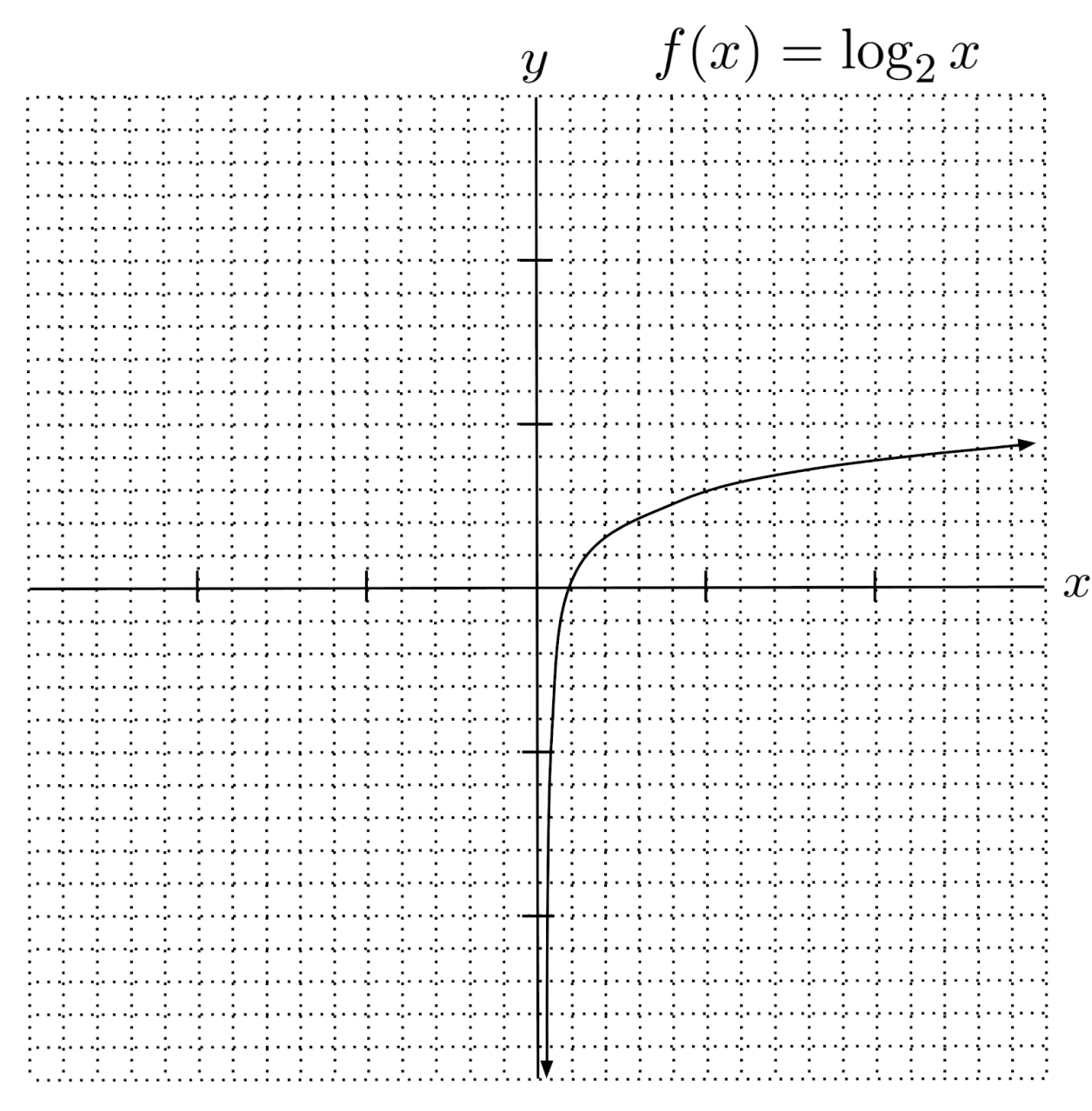Logarithmic graphs cross the x-axis at $1$ because raising any number to the power of $0$ results in $1$. That is, any logarithm $x=\log_b 1$ solves the equation $b^x=1$, which we already know is solved by $x=0$.

Also, logarithmic graphs extend to negative infinity as $x$ approaches $0$, because a number (greater than one) gets smaller and smaller as its exponent gets more and more negative.

$\begin{matrix} 2^{-1}=\frac{1}{2} &\hspace{.5cm}& 2^{-2}=\frac{1}{4} &\hspace{.5cm}& 2^{-3}=\frac{1}{8} \\ \log_2 \frac{1}{2} = -1 & & \log_2 \frac{1}{4} = -2 & & \log_2 \frac{1}{8} = -3 \end{matrix}$

Lastly, the base of the logarithm tells us where the y-value is $1$ – that is, the function $f(x)=\log_b x$ has $f(b)=1$. This is because $\log_b b$ is the exponent we have to raise $b$ to, to get $b$.

When the base of the logarithm is smaller than one, the graph flips over the x-axis.

In this case, the graph extends to positive infinity as $x$ approaches $0$, because a number smaller than $1$ gets closer and closer to $0$ as its exponent increases.

Likewise, as $x$ increases, the graph becomes more and more negative because a negative exponent is needed to flip the fractional base.

$\begin{matrix} \left( \frac{1}{2} \right)^1 = \frac{1}{2} &\hspace{.5cm}& \left( \frac{1}{2} \right)^2 = \frac{1}{4} &\hspace{.5cm}& \left( \frac{1}{2} \right)^3 = \frac{1}{8} \\ \log_\frac{1}{2} \frac{1}{2} = 1 & & \log_\frac{1}{2} \frac{1}{4} = 2 & & \log_\frac{1}{2} \frac{1}{8} = 3 \end{matrix}$

$\begin{matrix} \left( \frac{1}{2} \right)^{-1} = 2 &\hspace{.5cm}& \left( \frac{1}{2} \right)^{-2} = 4 &\hspace{.5cm}& \left( \frac{1}{2} \right)^{-3} = 8 \\ \log_\frac{1}{2} 2 = -1 & & \log_\frac{1}{2} 4 = -2 & & \log_\frac{1}{2} 8 = -3 \end{matrix}$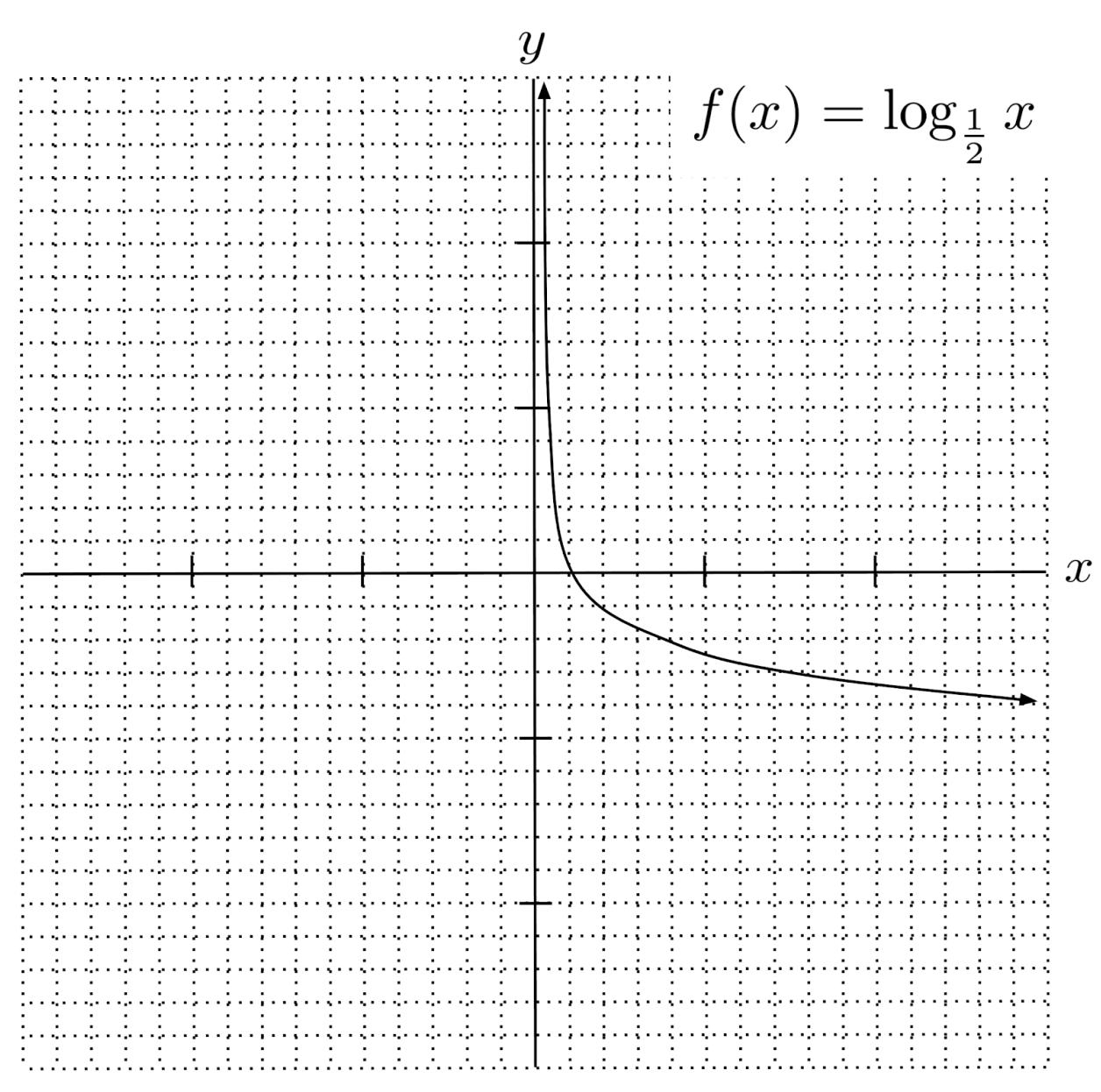## Properties of Logarithms

Expressions consisting of multiple logarithms of the same base can be simplified by using two properties of logarithms:

1. Addition outside two logarithms with the same base turns into multiplication inside a single logarithm. For example, $\log_2 4 + \log_2 8 = \log_2 32$, and in general, $\log_b x + \log_b y = \log_b xy$.
2. Multiplication outside two logarithms with the same base turns into exponentiation inside a single logarithm. For example, $3\log_2 4 = \log_2 4^3 = \log_2 64$, and in general, $a \log_b x = \log_b x^a$.

A particularly noteworthy consequence of the second rule is that negative outside a log turns into reciprocal inside the log:

$-\log_b x = (-1) \log_b x = \log_b x^{-1} = \log_b \dfrac{1}{x}$

Sometimes, logarithms of different bases can be converted to logarithms of the same base. For example, $\log_2 4$ is the same as $\log_4 16$. In general, $log_{b^n} x^n = \log_b x$ provided both logarithms exist.

Below is an example of simplifying a logarithmic expression using all of the properties that we have discussed:

\begin{align*} \text{Original expression} \hspace{.5cm} &\Bigg| \hspace{.5cm} \log_2 x - \log_4 x \\ \text{Rewrite using addition} \hspace{.5cm} &\Bigg| \hspace{.5cm} \log_2 x +(-1) \log_4 x \\ \begin{matrix} \text{Convert multiplication} \\ \text{to exponentiation} \end{matrix} \hspace{.5cm} &\Bigg| \hspace{.5cm} \log_2 x + \log_4 x^{-1} \\ \text{Simplify} \hspace{.5cm} &\Bigg| \hspace{.5cm} \log_2 x + \log_4 \frac{1}{x} \\ \begin{matrix} \text{Square base and} \\ \text{argument} \end{matrix} \hspace{.5cm} &\Bigg| \hspace{.5cm} \log_{2^2} x^2 + \log_4 \frac{1}{x} \\ \text{Simplify} \hspace{.5cm} &\Bigg| \hspace{.5cm} \log_4 x^2 + \log_4 \frac{1}{x} \\ \begin{matrix} \text{Convert addition} \\ \text{to multiplication} \end{matrix} \hspace{.5cm} &\Bigg| \hspace{.5cm} \log_4 \left( x^2 \cdot \frac{1}{x} \right) \\ \text{Simplify} \hspace{.5cm} &\Bigg| \hspace{.5cm} \log_4 x \\ \end{align*}

## Exercises

Graph the following exponential functions. (You can view the solution by clicking on the problem.)

$1) \hspace{.5cm} f(x)=3^x$
Solution: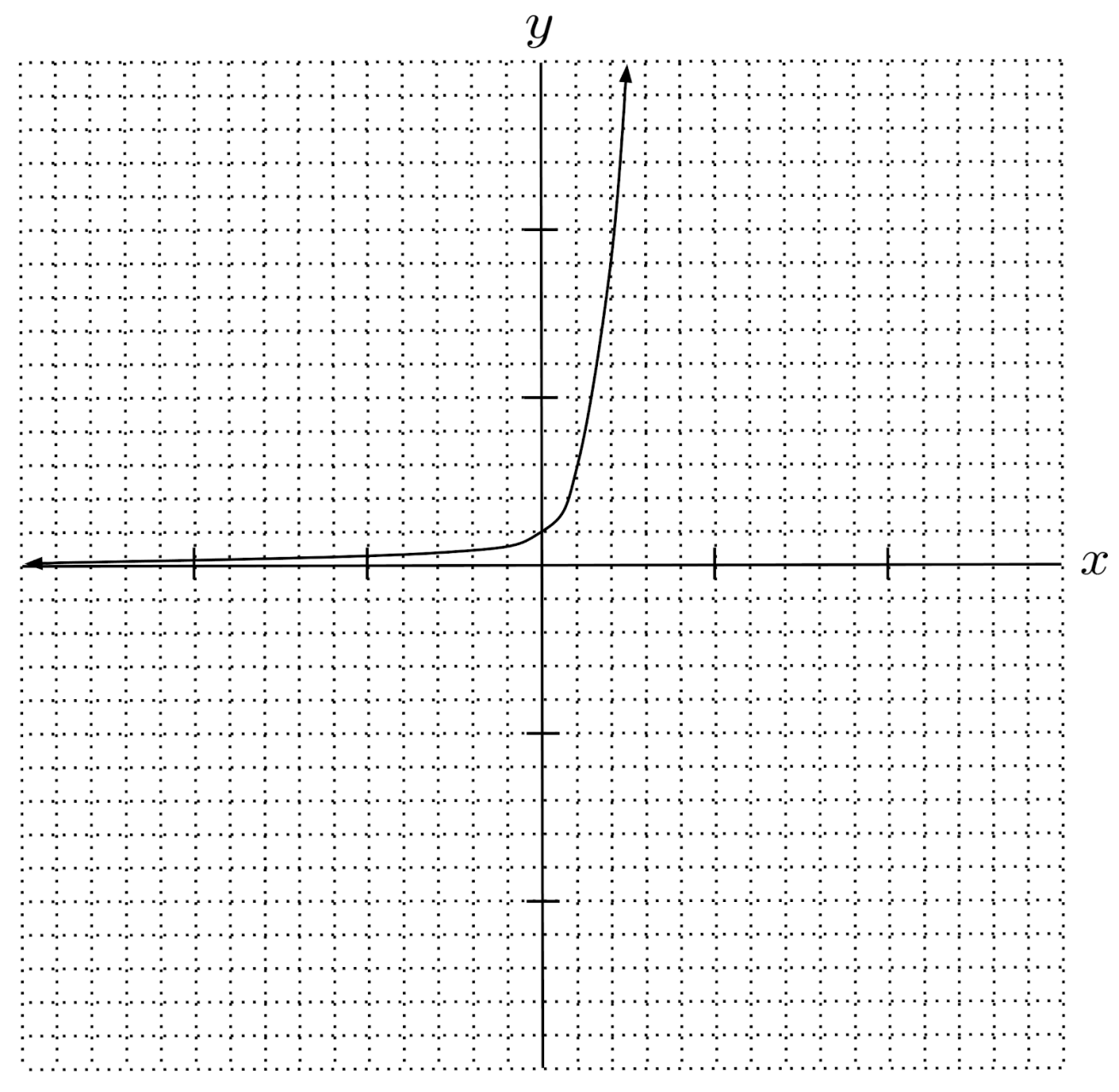$2) \hspace{.5cm} f(x)=5^x$
Solution: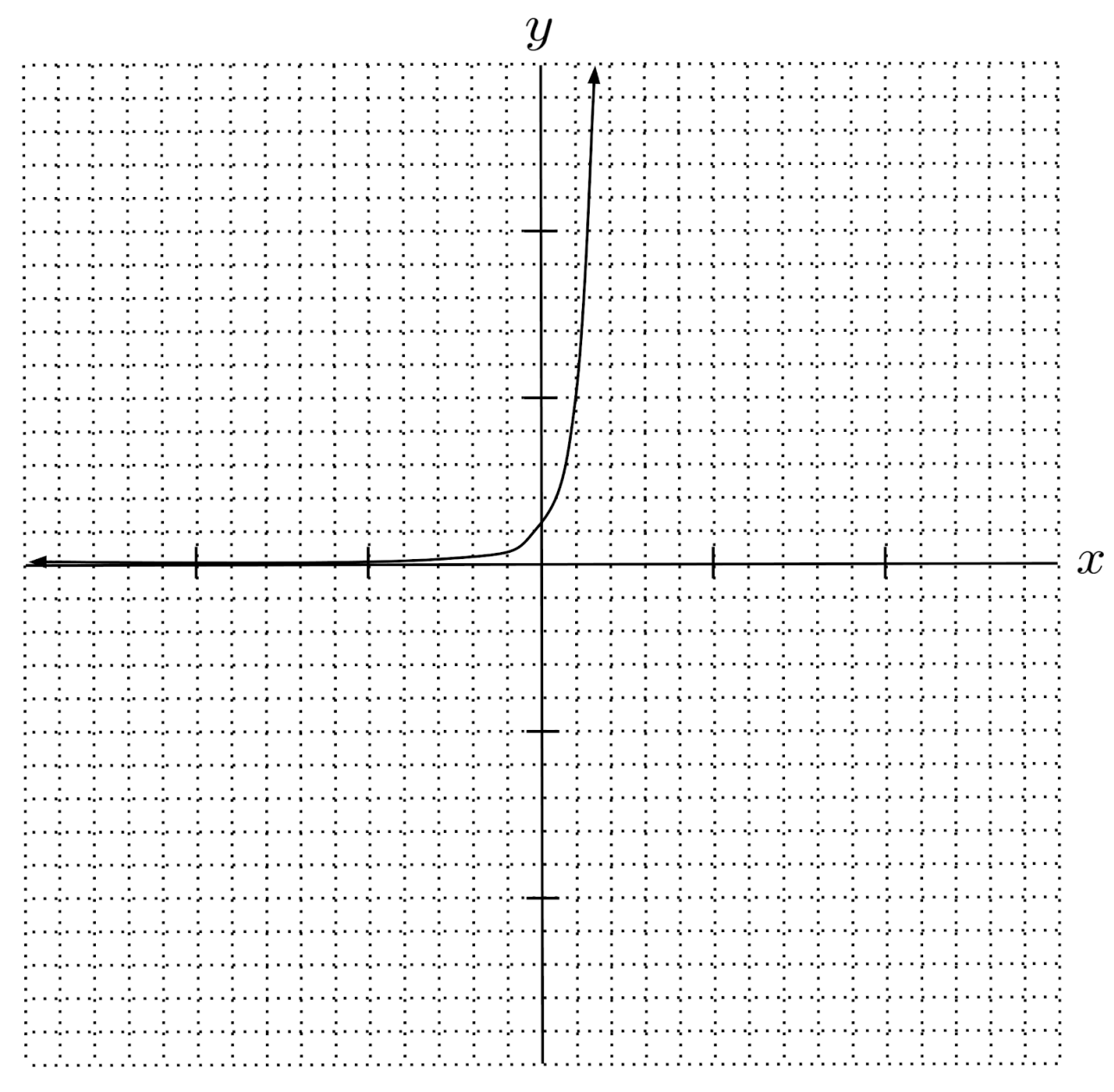$3) \hspace{.5cm} f(x)= \left( \frac{1}{3} \right)^x$
Solution: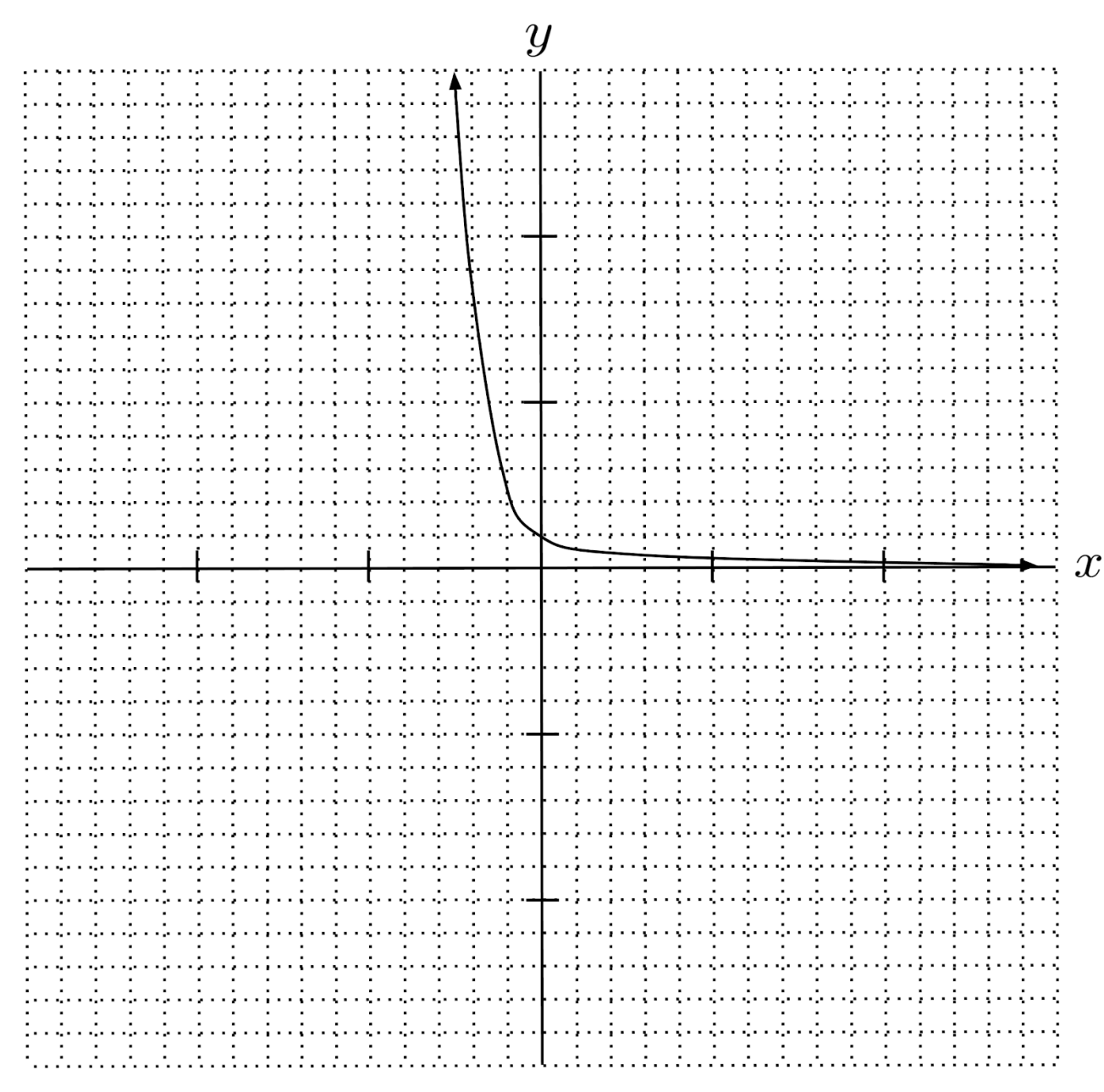$4) \hspace{.5cm} f(x)= \left( \frac{1}{5} \right)^x$
Solution: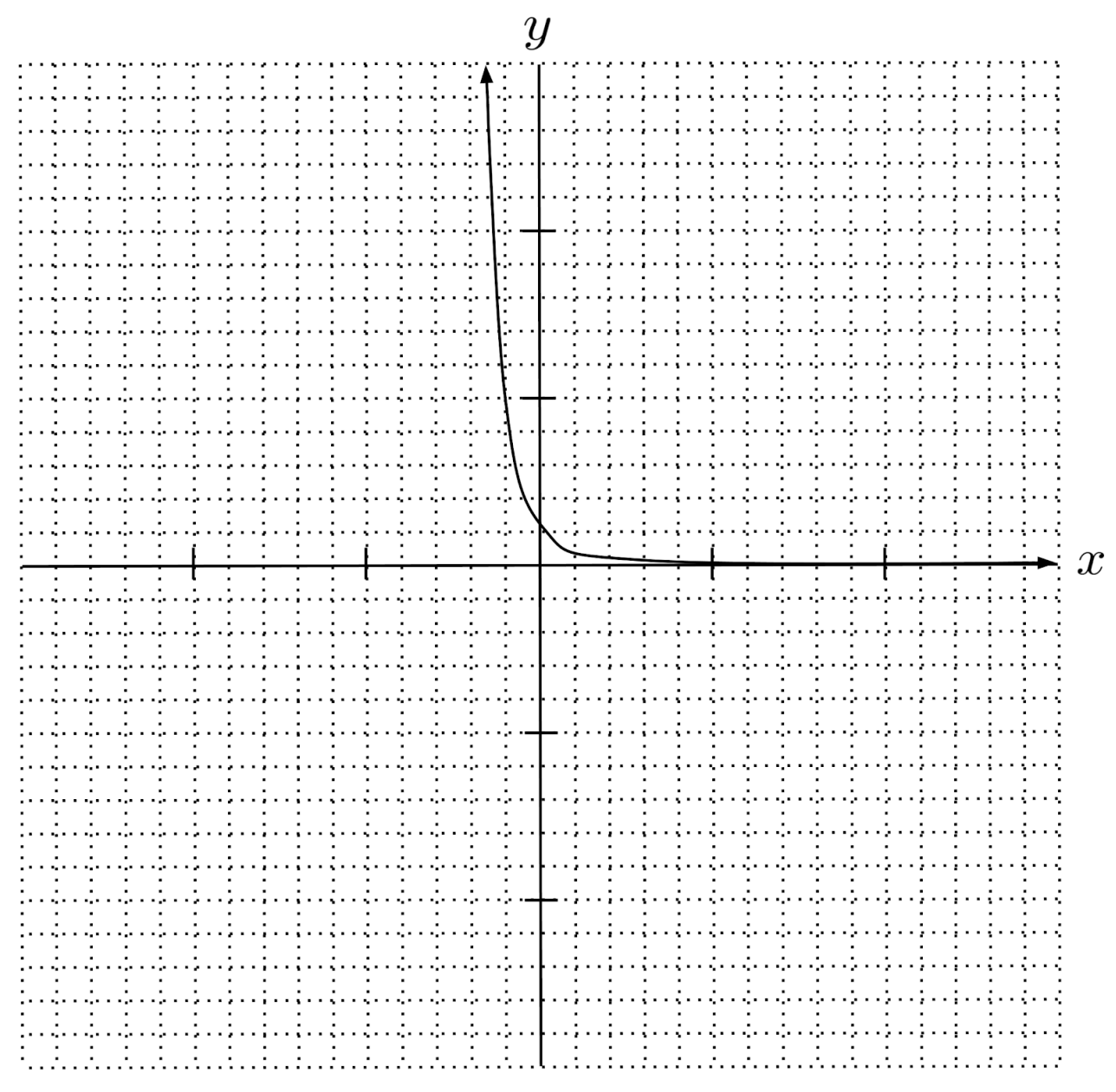$5) \hspace{.5cm} f(x)= \left( \frac{3}{2} \right)^x$
Solution: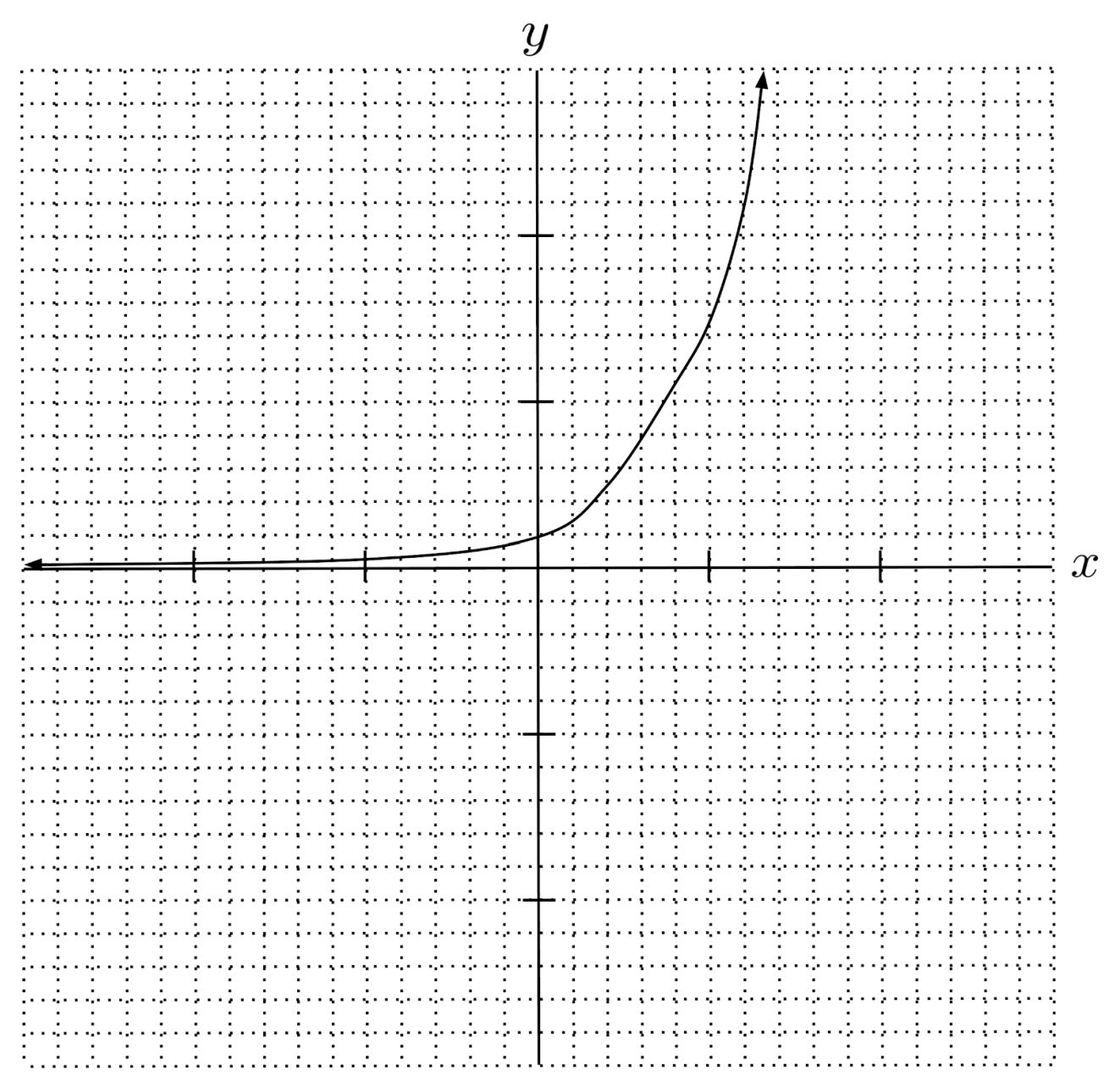$6) \hspace{.5cm} f(x)= \left( \frac{2}{3} \right)^x$
Solution: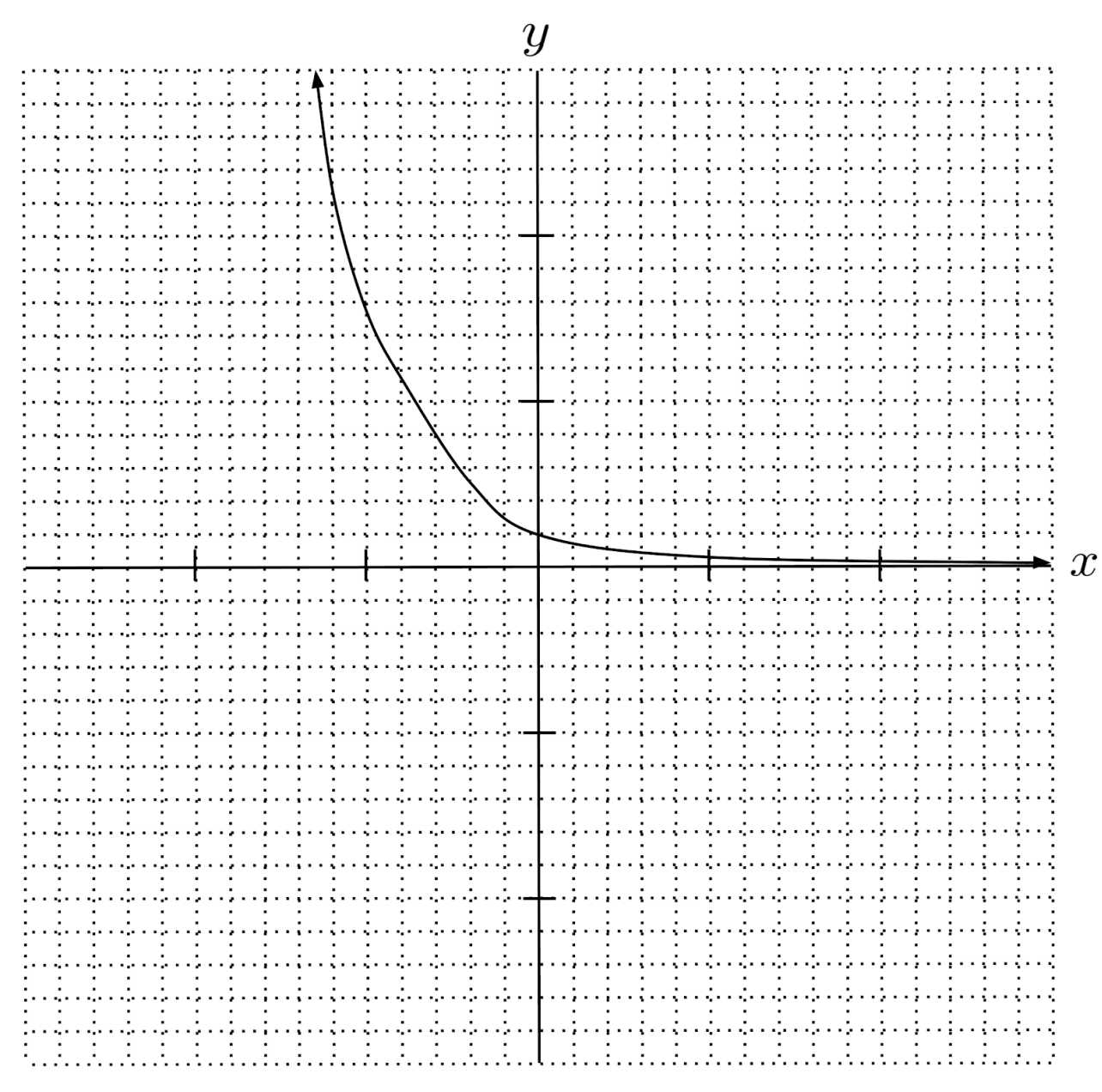Use logarithms to solve the following exponential equations. (You can view the solution by clicking on the problem.)

$7) \hspace{.5cm} 3^x=10$
Solution:
$\log_3 10 \approx 2.096$

$8) \hspace{.5cm} 5^x=7$
Solution:
$\log_5 7 \approx 1.209$

$9) \hspace{.5cm} \left( \frac{1}{3} \right)^x=\frac{1}{10}$
Solution:
$\log_{\frac{1}{3}} \frac{1}{10} \approx 2.096$

$10) \hspace{.5cm} \left( \frac{1}{5} \right)^x = \frac{1}{2}$
Solution:
$\log_{\frac{1}{5}} \frac{1}{2} \approx 0.431$

$11) \hspace{.5cm} \left( \frac{3}{2} \right)^x = 9$
Solution:
$\log_{\frac{3}{2}} 9 \approx 5.419$

$12) \hspace{.5cm} \left( \frac{2}{3} \right)^x = \frac{1}{5}$
Solution:
$\log_{\frac{2}{3}} \frac{1}{5} \approx 3.969$

Graph the following logarithmic functions. Use logarithm rules to simplify the expression, if needed. (You can view the solution by clicking on the problem.)

$13) \hspace{.5cm} f(x)=\log_3 x$
Solution:
$f(x) = \log_3 x$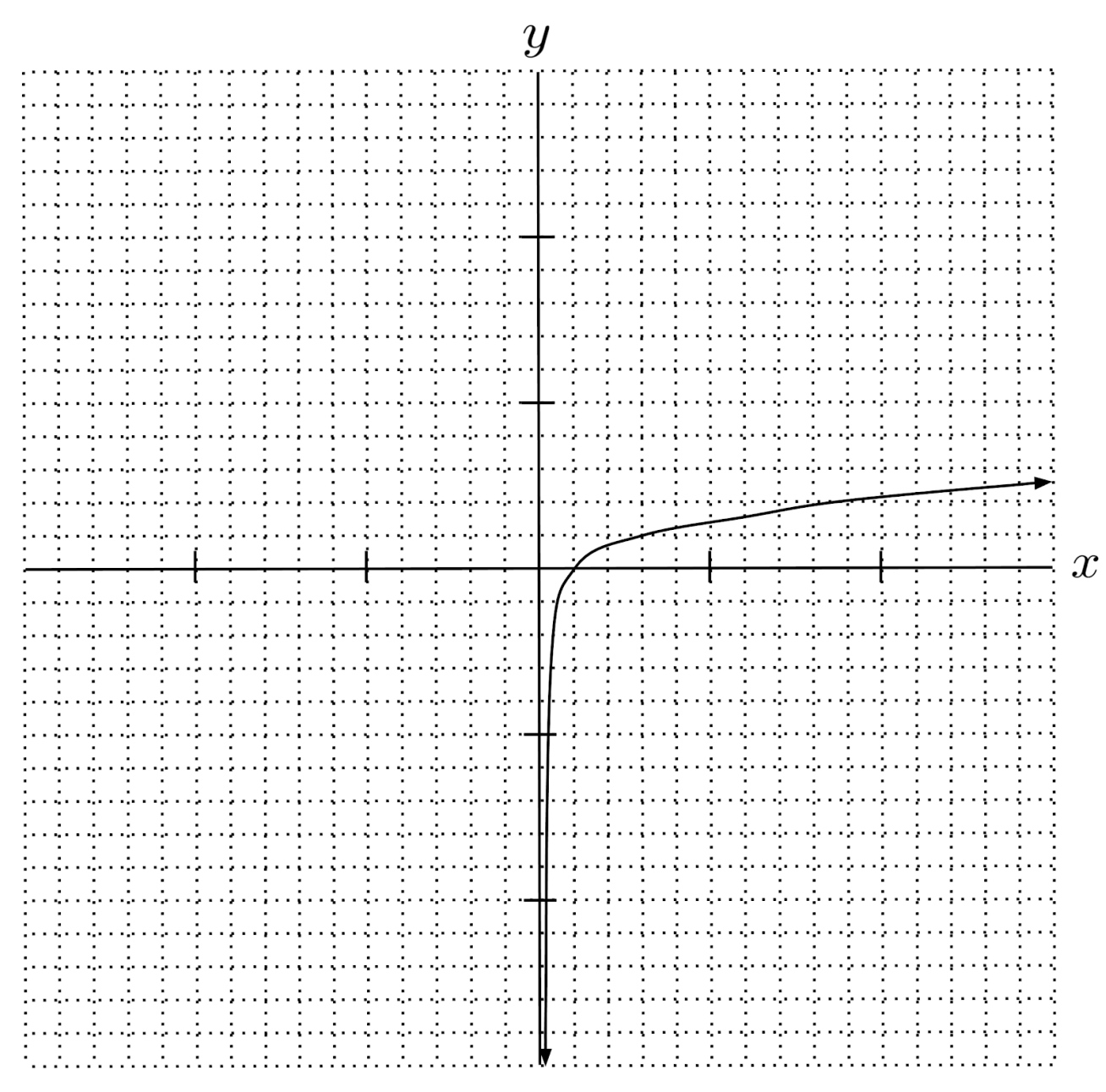$14) \hspace{.5cm} f(x)= \log_5 x$
Solution:
$f(x) = \log_5 x$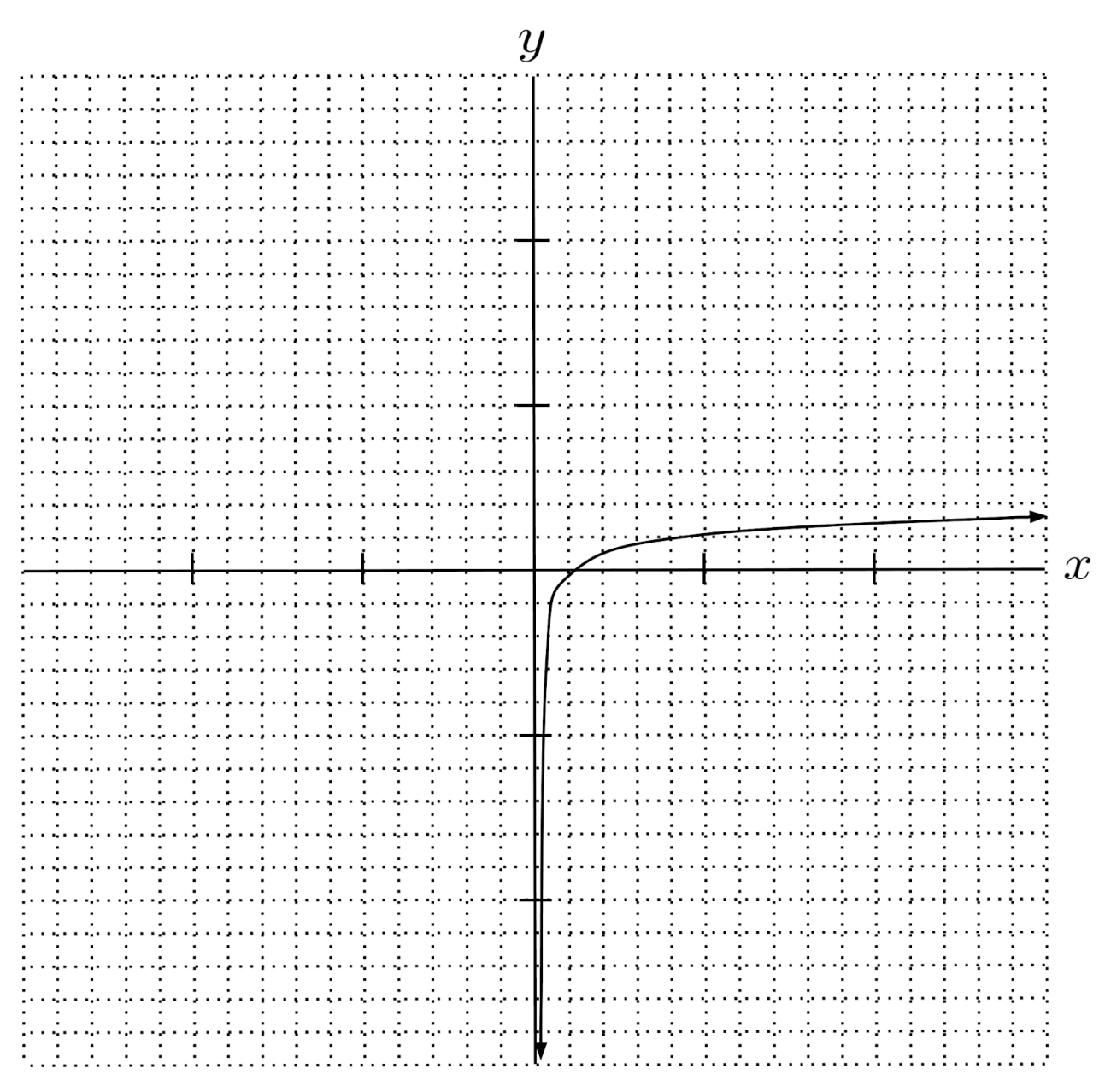$15) \hspace{.5cm} f(x)=\log_{\frac{1}{3}} x$
Solution:
$f(x) = \log_\frac{1}{3} x$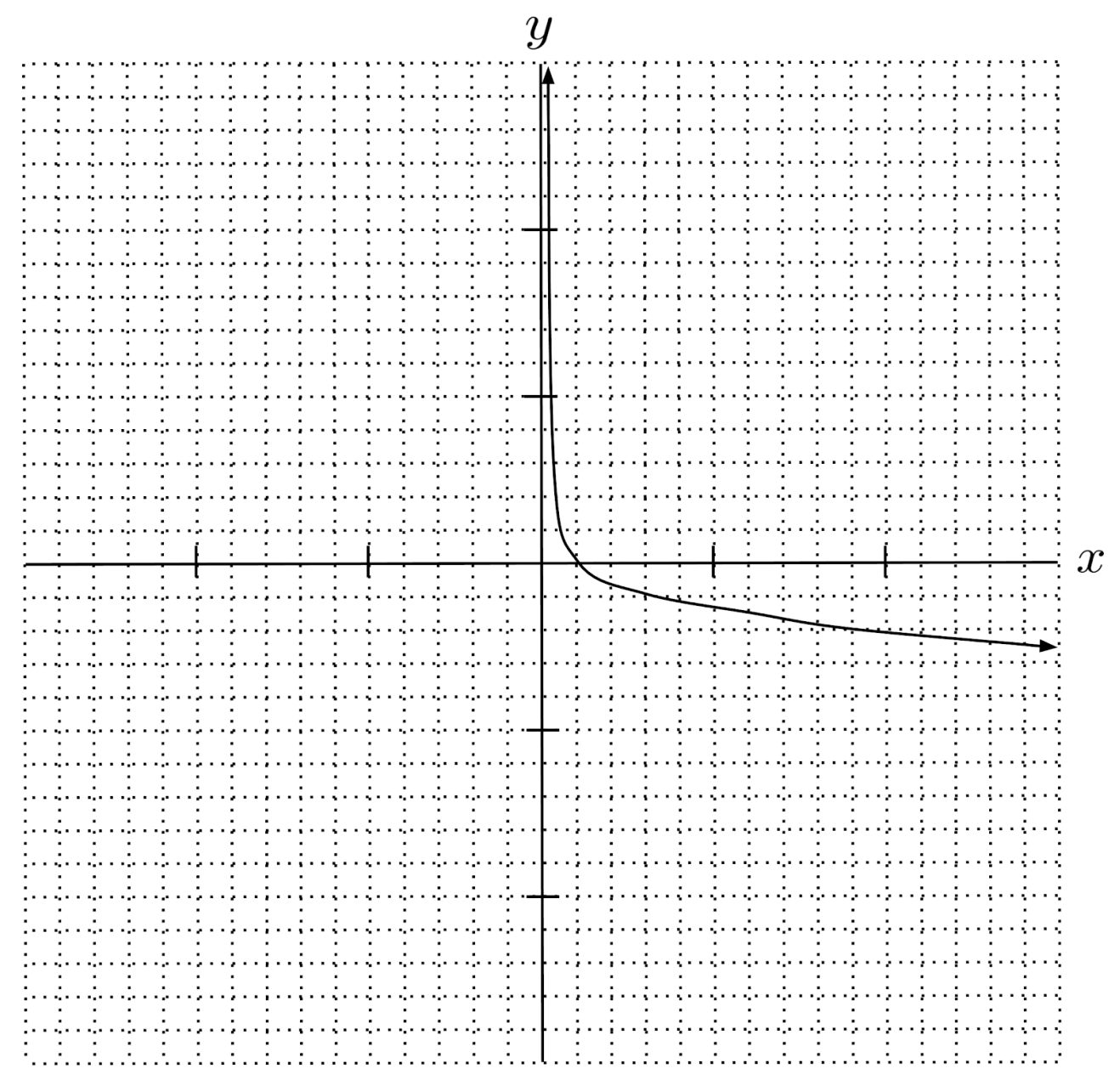$16) \hspace{.5cm} f(x)=\log_{\frac{1}{5}} x$
Solution:
$f(x) = \log_\frac{1}{5} x$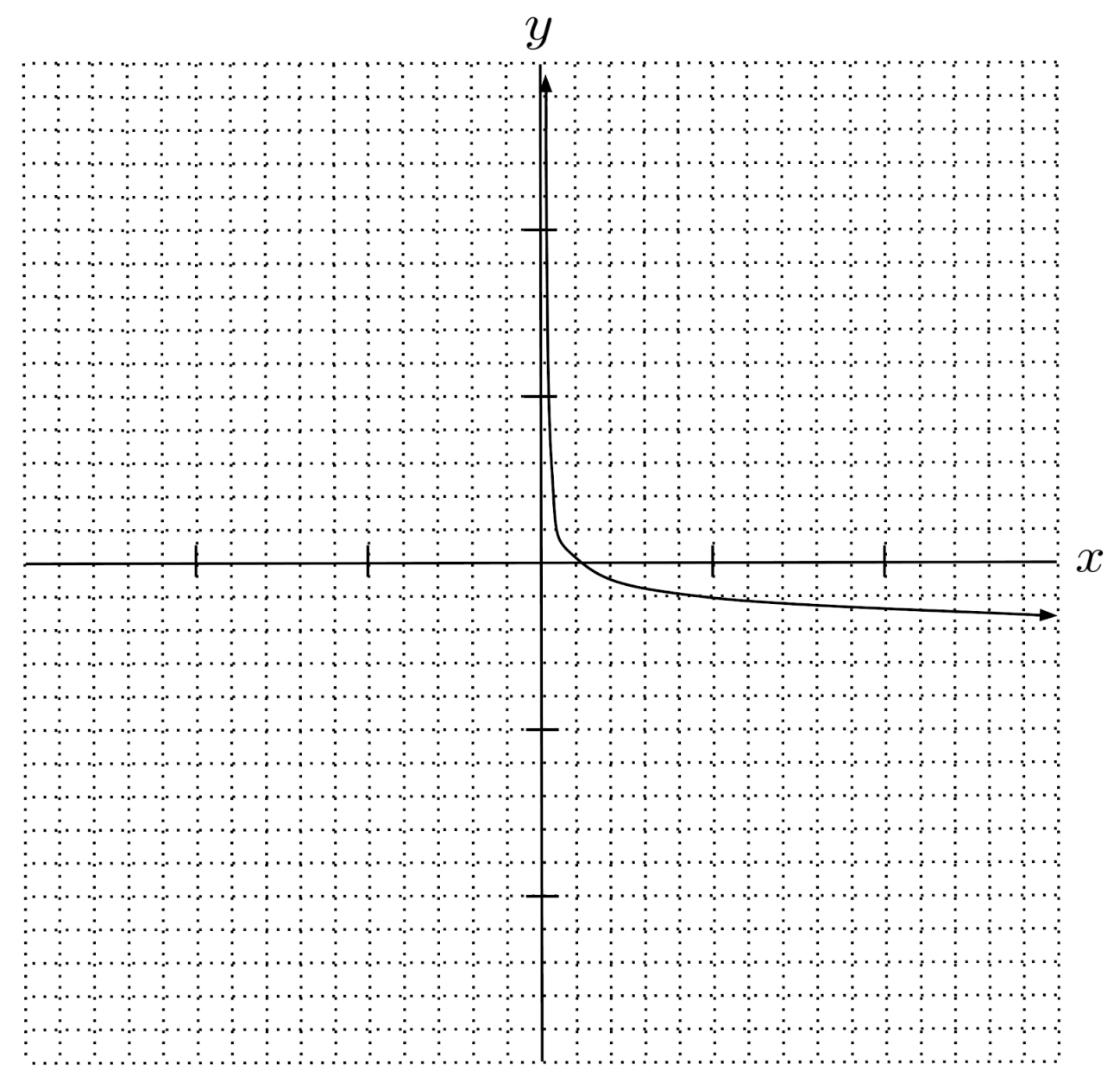$17) \hspace{.5cm} f(x)= \log_2 \sqrt{x}$
Solution:
$f(x) = \log_4 x$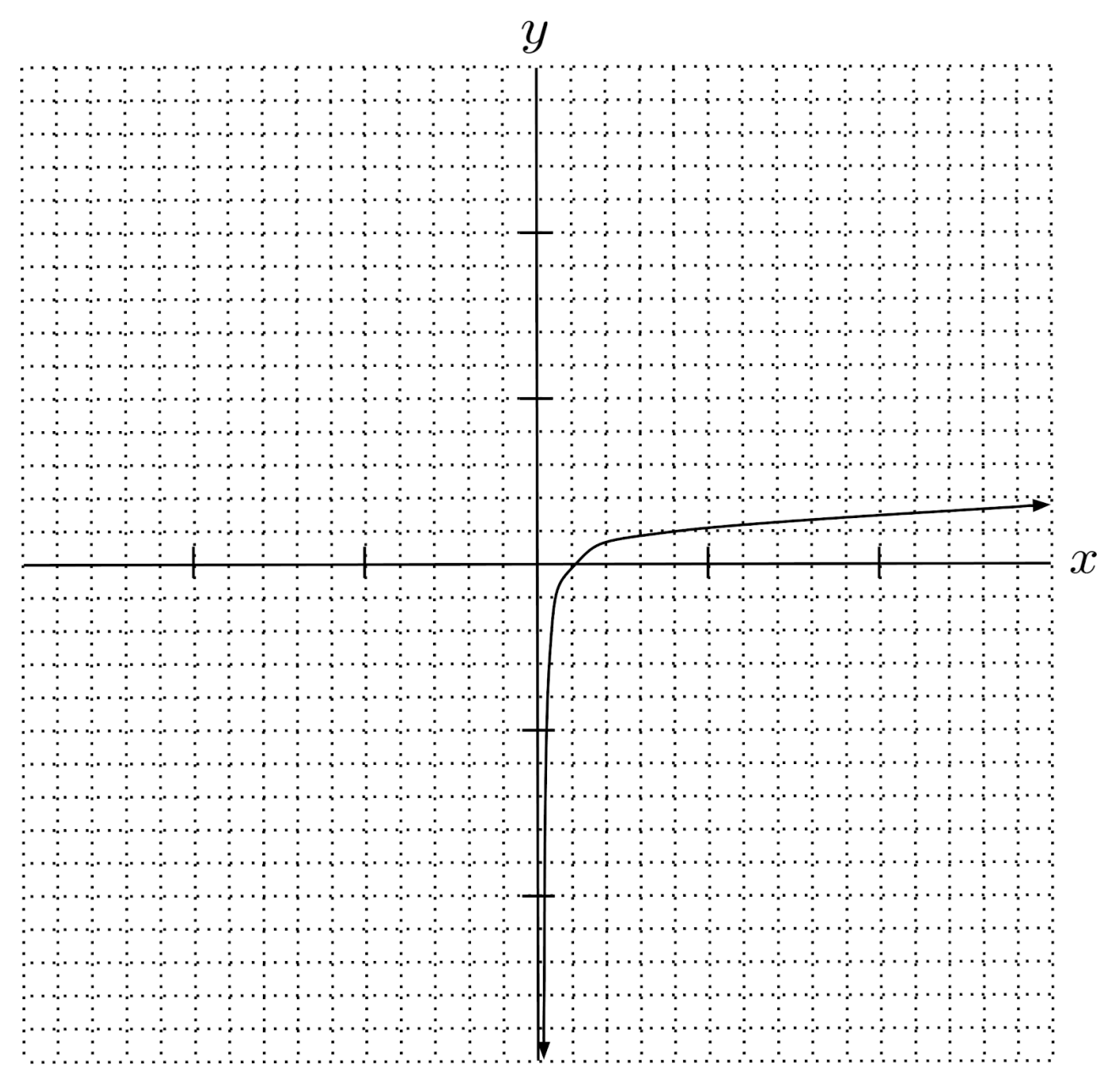$18) \hspace{.5cm} f(x)= \log_{10} x^2 - \log_{10} x$
Solution:
$f(x) = \log_{10} x$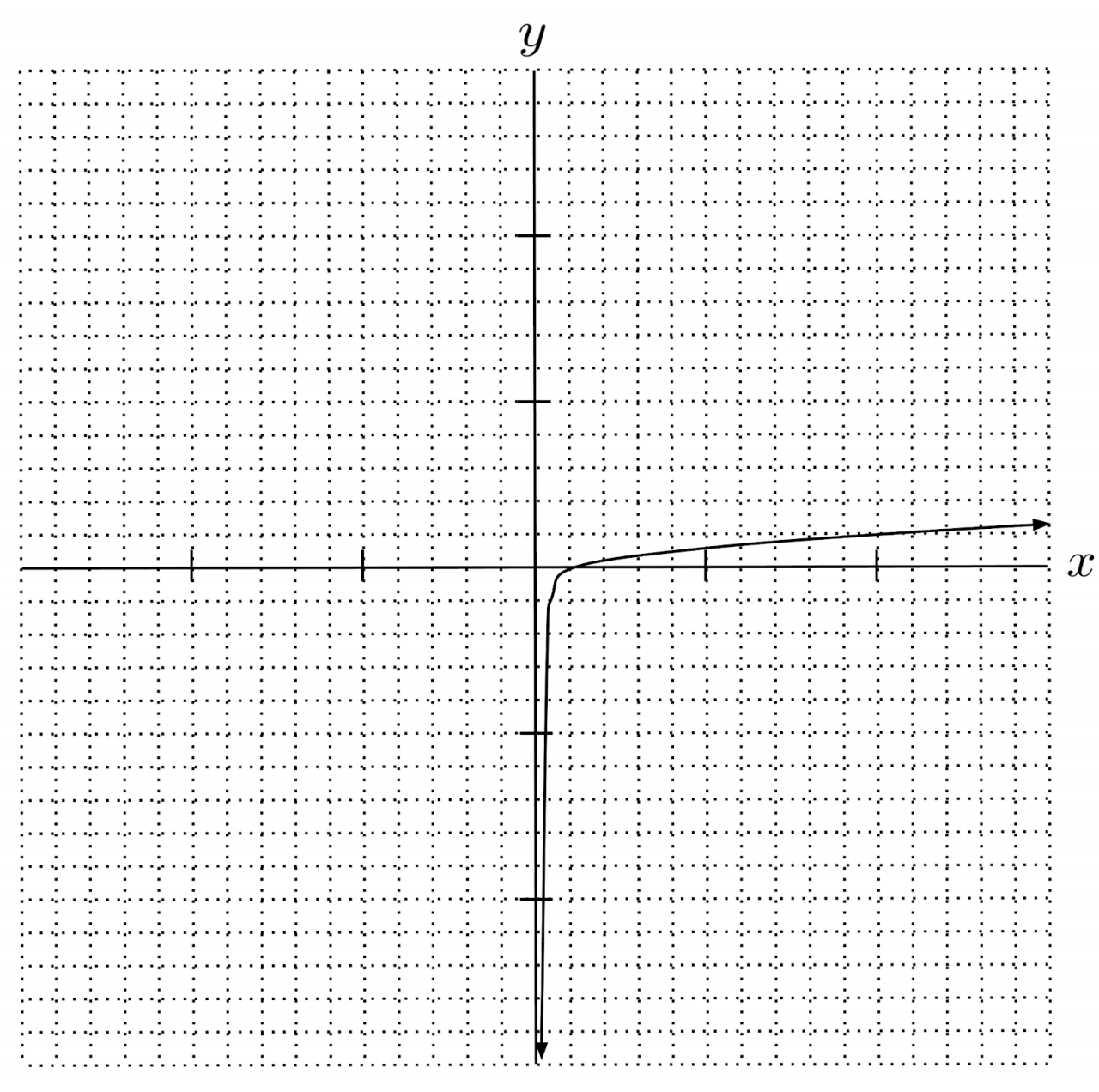$19) \hspace{.5cm} f(x)=3 \log_3{1} + \frac{1}{2} \log_3{x}$
Solution:
$f(x) = \log_{9} x$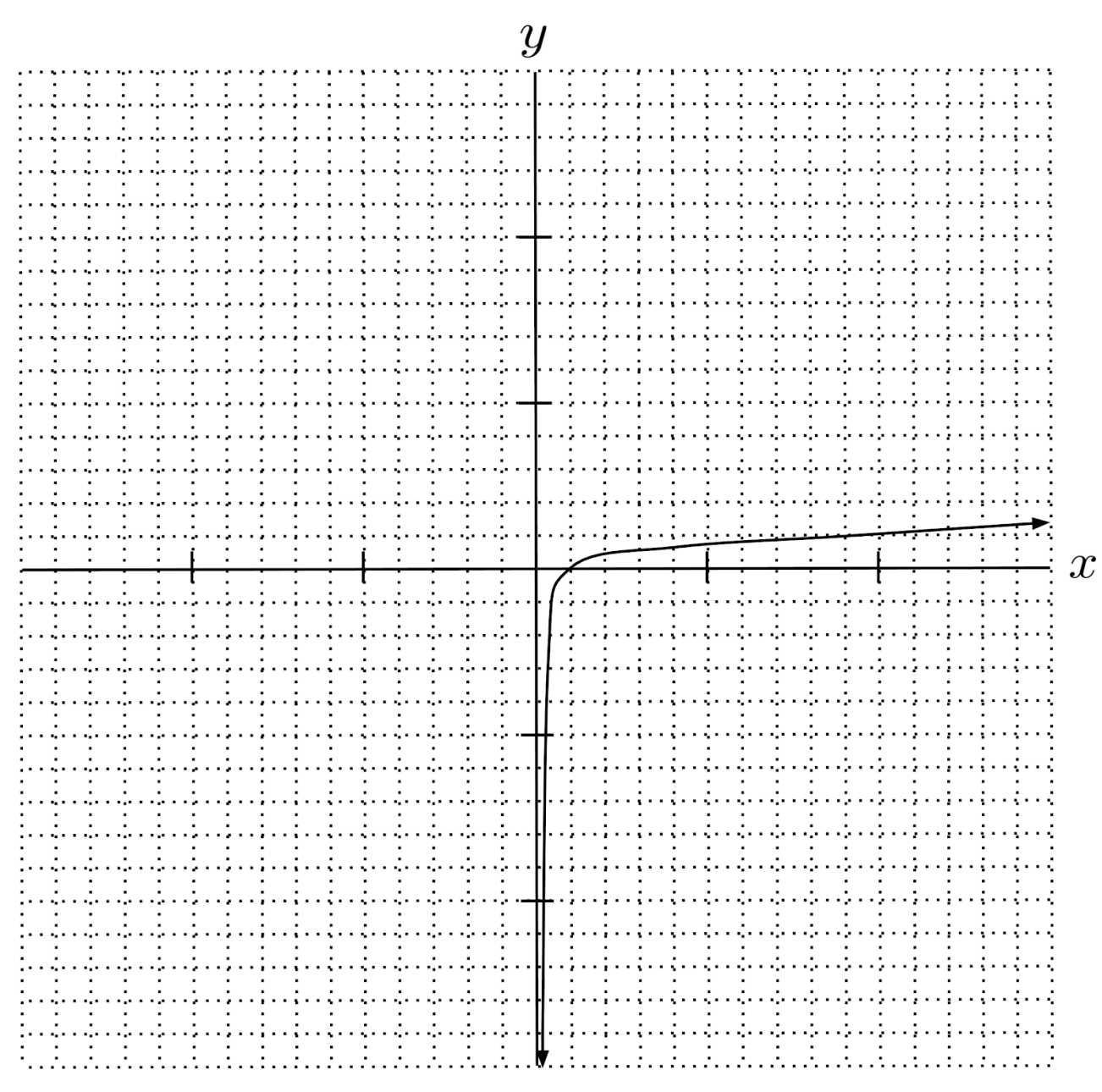$20) \hspace{.5cm} f(x)=\log_2{x} - 2\log_4 \sqrt{x}$
Solution:
$f(x) = \log_4 x$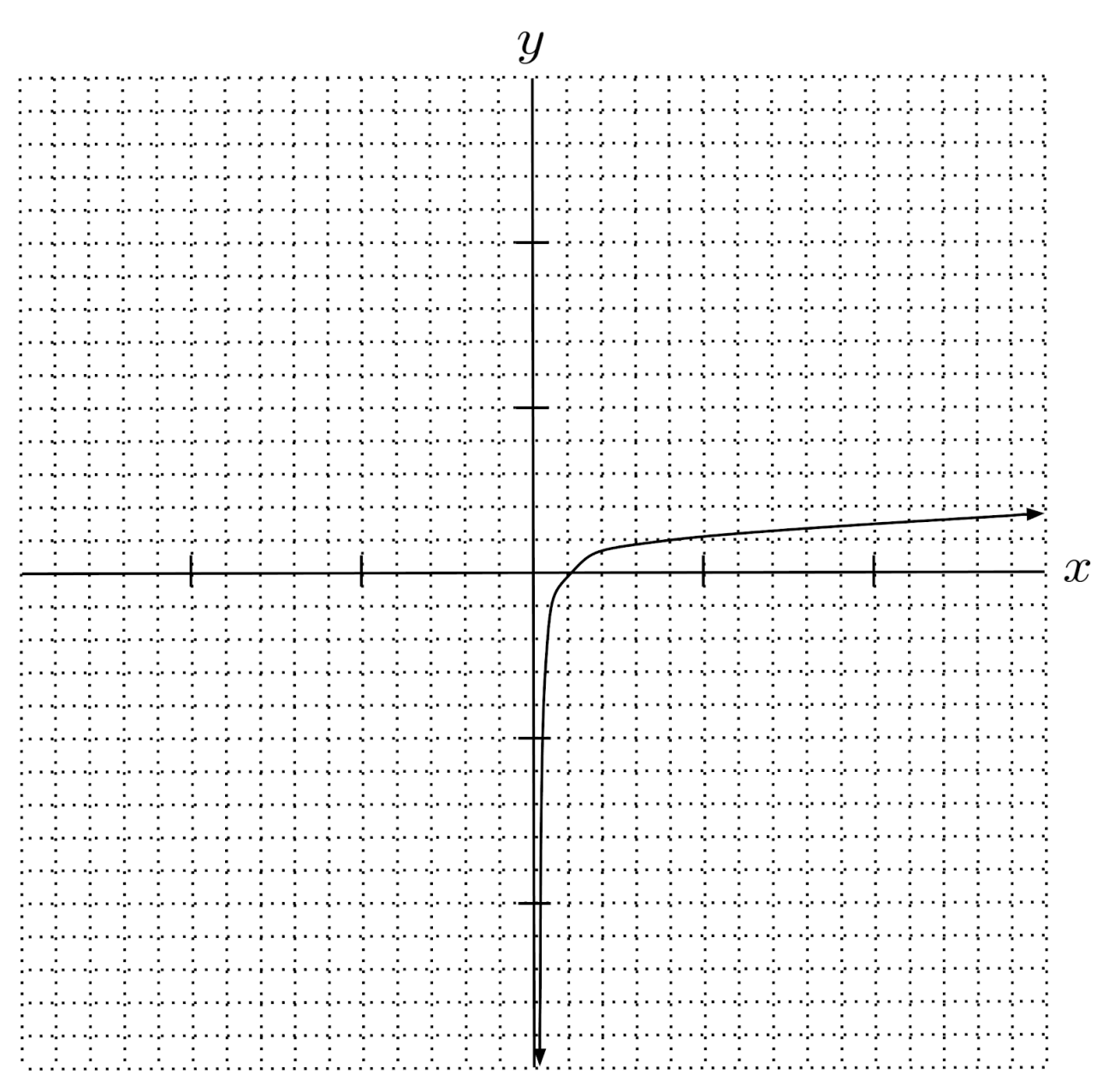This post is part of a series.

Tags: